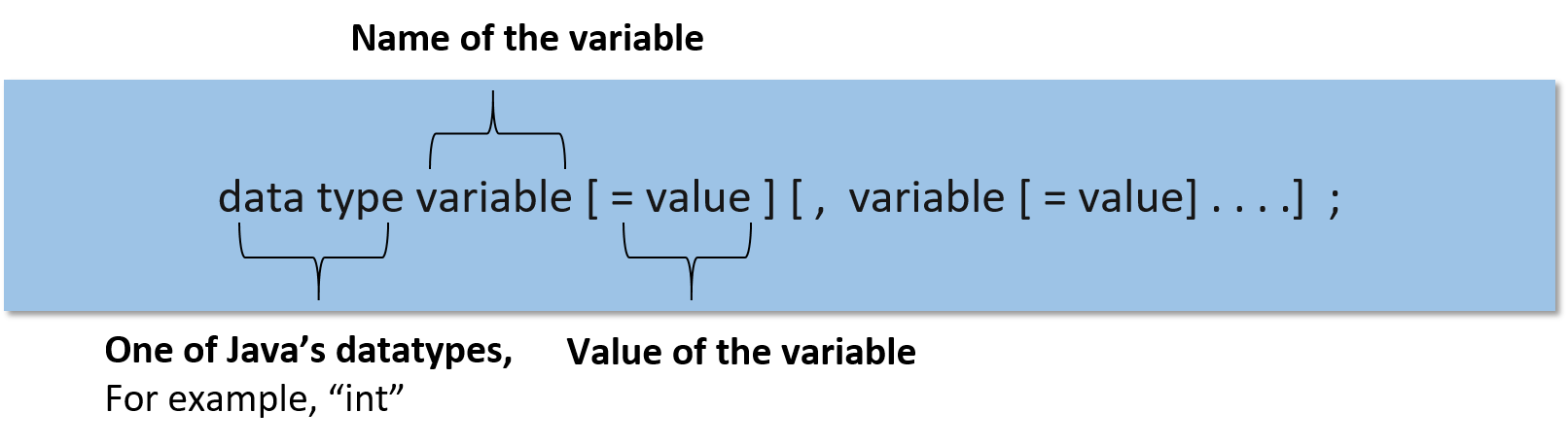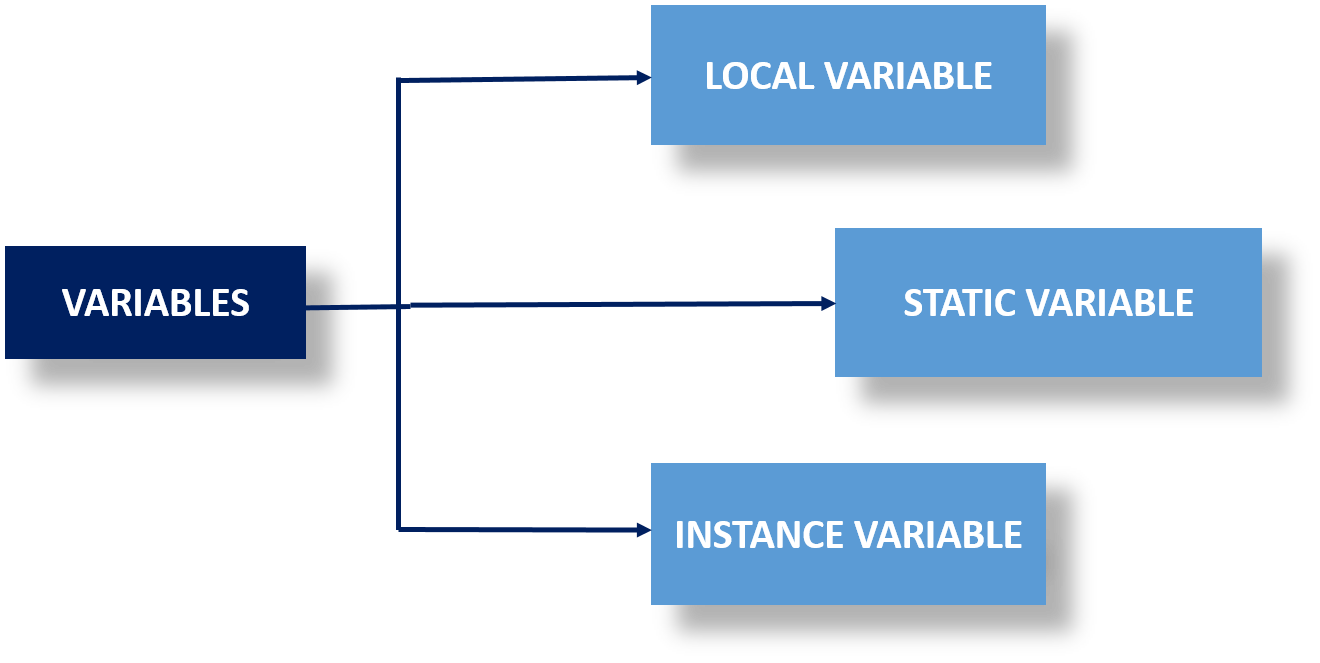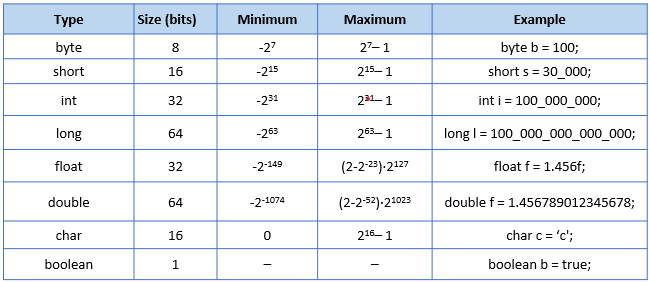# Java Variables and Data Types Explained

07 Feb 2023
Beginner
349 Views

## Java Variables and Data Types Explained

Java is an "object-oriented", "network-centric", and "multi-platform" based program language that is widely used for fast, reliable, and secure coding for web development. This computing platform is designed for experiencing lesser dependency to implement the language. Java applications are vastly used in cell phones, laptops, scientific supercomputers, and developing video games.

### What is a Variable in Java?

Variables work as a container to store the values of data. It saves the data while the language is executing a program. Every Variable has a data type that understands and designates the quantity and type of the value that can be stored by the container. It is a memory location that memorizes the location of the data.

### Variable Declaration

• There are various types of declaration that happen by using java variables such as
• Strings- which stores text, Such as "Hello world".
• Int- it stores any integer, specifically any whole number without decimal.
• Float- stores "floating point" numbers that include decimal values
• Char- it stores characters such as 'x', and 'y'. It denotes by single quotes
• Boolean- store values if true or false state.
To declare the variable programmers, need to focus on two things, are
1. datatype: it denotes the types of Data that are going to store
2. data_name: a particular name of that variable
``````class Example
{
public static void main ( String[] args )
{
long payAmount = 184; //the declaration of the variable

System.out.println("The variable contains: " + payAmount );
}
}
``````
This program is an example of using the variable "payAmount".
Output
Long payAmount = 184;

### Variable Initialization

For initializing the variable there are 3 major components, are
• datatype: it defines what type of data is going to store in a variable
• variable_name: Particular name of the variable
• value: initially stored value in the variable### Types of variables in Java

There are three types of variables in Java:#### Local variables

• It is created when a block is entered in the storage and then it calls and destroys the block just after exiting from the function.
• It is important to initialize a local variable before using it.
``````import java.io.*;

class DNT
{
public static void main(String[] args)
{
int var = 89; // Declared a Local Variable

// This variable is local to this main method only

System.out.println("Local Variable: " + var);
}
}
``````
Output:
Local Variable: 89

#### Instance Variables

• An instance variable is created when an object class is generated and it gets destroyed when the same object class is destroyed
• Programmers can use access specifiers for instance variables
• It is not mandatory to initialize instance variables
``````import java.io.*;

class DNT
{
public String student; // Declared Instance Variable
public DNT()
{ // Default Constructor
this.student= "Urmi Bose"; // initializing Instance Variable
}
//Main Method
public static void main(String[] args)
{
// Object Creation
DNT name = new DNT();
System.out.println("Student name is: " + name.student);
}
}
``````
Output:
Student name is : Urmi Bose

#### Static variables

• Static variables are declared as instance variables.
• It is created to start the execution of the program and then destroy it automatically after the execution ends.
• It uses static keywords for the declaration of the static variable within a class
``````import java.io.*;
class DNT
{
public static String student= "Urmi Bose"; //Declared static variable

public static void main (String[] args) {

//student variable can be accessed without object creation
System.out.println("Student Name is : "+DNT.student);
}
}
``````
Output:
Student Name is : Urmi Bose

### Data Types in Java

Data types in Java describe the various sizes and values which can be stored in that particular variable. The data types of Java are divided into two groups
1. "Primitive" data type- this particular data type includes float, short, boolean, byte, char, long, int, and double.
2. "Non-primitive" data type- this particular data type includes arrays, interfaces, strings, and classes.

### Primitive Data Type in Java

The primitive data type is primarily a set of primary data from which other data and data types could be constructed. Primitive data refers to some limited set of data that is represented by an individual processor. So that all the compiled programs could use this data type. Primitive data is already defined in Java and does not need to be created by programmers. There are 8 types of primitive data which will be discussed further.

#### Float data type

This particular data type has a "single precision 32 bits IEEE 754" floating point. The value of this range is unlimited. In contemplation of saving memories, one should use the "Float" data type as it saves memories in a large array of that floating number.

#### Short data type

This data type is a "16-bit" data type which is a two's complement integer. The value range of the Short data type stands between "-32,768 to 32,767". So the lowest value is -32,768 and the highest value is 32,767 and the default value is 0. This data type can also be applied to save the memory just like the byte data type. Also, the short data type is "2 times smaller" than an integer data type.

#### Boolean data type

This primitive data type identifies "one bit of information" but the size of the particular data type could not be defined precisely. Boolean data type stores only two possible outcomes of an input. Either the value could be true or it could be false. This data type is generally used to track whether the conditions are true or false.

#### Byte data type

Byte-data type helps to save the memories in a large array according to the requirements of memory saving. This is an "8 bits two's complement integer". The value range stands between -128 to 127. The lower range is -128 and the upper range is 127 and 0 is the default value of this data type. Byte data type helps to save space because it is 4 times smaller than integer data type.

#### Char data type

The char data type stands for a character data type which is a "single 16-bit Unicode" character. The range of the data types lies between 0 to 65,535. This particular data type helps to store the character in the database.

#### Long data type

This 64-bit long data type is a two's complement integer. The minimum value of the long data type is 9 223,372 036 854,775 808 and the maximum value of the long data type is 9,223,372,036,854,775,807. The default value of this long data type is 0. The long data type is specifically used when the user needs a much bigger value range than an integer.

#### Int data type

The int data type stands for integer data type and it is a "32-bit signed two's complement integer". The minimum value of the int data type is 2,147,483,648 and 0 is the default value. Int data type is a default data type that is used for integral values.

#### Double data type

This particular data type is a "64-bit Double precision IEEE 754 floating point". The value range of the Double data type is unlimited. Double data type helps to use decimal values like a float. As this data type does not have any particular values so this cannot generate precise values such as "currency".### Non-Primitive Data Type in Java

Non-primitive data type depends on the object. The value of a variable in the Non-primitive data could not be stored directly in the memory. This particular data stores the address of the memory of the variable. Non-primitive data has to be defined by its user. There are 4 types of non-primitive data which will be discussed shortly.

#### Arrays

An array is a container that can only hold a particular number of values of a significant type of data. This user-defined data type generally establishes its length at the time of its creation. After that, it was fixed and could not change. It stores multiple values in a single and particular variable.

#### Interfaces

The interface is a descriptive reference type in Java that is used for describing the actions and behavior of a particular object. Interfaces are the collection of "abstract methods". To achieve abstraction Java follows an interface mechanism. Interface data type contains "constants", "default methods", "static methods" and "nested type".

#### Strings

Strings are non-primitive data types that represent a particular number of the values of a character. Strings are represented by various alphabets and represented in a sequence of order. For example, strings are represented as char[ ] where ch stands for { 'j', 'a', 'v', 'a'}.

### Summary

This article covers the definition of the variable, variable declaration, and variable initialization. It also generates a vast idea of different types of variables and various data types.